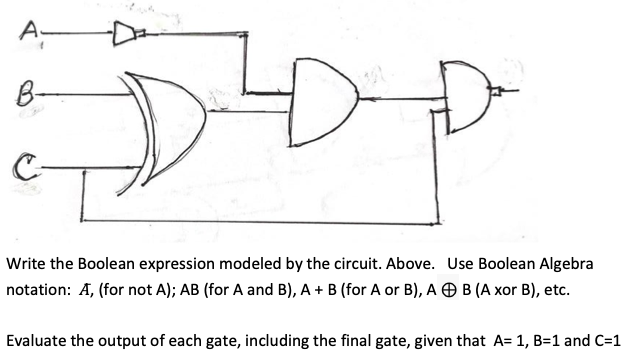# A-B-Write the Boolean expression modeled by the circuit. Above. Use Boolean Algebranotation: A, (for not A); AB (for A and B), A + B (for A or B), A O B (A xor B), etc.Evaluate the output of each gate, including the final gate, given that A= 1, B=1 and C=1

Question
53 viewshelp_outlineImage TranscriptioncloseA- B- Write the Boolean expression modeled by the circuit. Above. Use Boolean Algebra notation: A, (for not A); AB (for A and B), A + B (for A or B), A O B (A xor B), etc. Evaluate the output of each gate, including the final gate, given that A= 1, B=1 and C=1 fullscreen
check_circle

Step 1

The given circuit has:

• A XOR gate between B and C.
• A AND gate between output of previous gate and output of NOT gate with A.
• A NAND gate between the output of ...

### Want to see the full answer?

See Solution

#### Want to see this answer and more?

Solutions are written by subject experts who are available 24/7. Questions are typically answered within 1 hour.*

See Solution
*Response times may vary by subject and question.
Tagged in
EngineeringComputer Science

### Boolean Logic